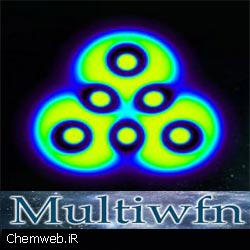September 24, 2021|Multiwfn software free download is a multifunctional realizing electronic wavefunction analyzer in computational chemistry. Multiwfn is free, open-source, high-efficient, very user-friendly and flexible, it supports almost all of the most important wavefunction analysis methods.

Key features of Multiwfn software:

• Ability to read wave function from input file types such as wfn, wfx, fch, NBO plotfile, Molden input file and read file structure from pdb, xyz, DMOL3, grd, gaussian cub files
•  analysis using different theories: Mayer bond order, multi-center bond order and…
• Topology analysis for electron density (AIM analysis)
• Population analysis using different methods: Hirshfeid, VDD, Muliken, Luwdin, Modified MPA and…
• Drawing UV-vis / ECP, RAMAN, IR, VCD spectra
• Display of molecular structure and orbitals, MO, NBO, natural orbitals, and…
• Direct display of results in three-dimensional structure, graphs and charts
• Calculate many parameters that can be extracted from wave functions
• Calculate many virtual indices dependent on wave function such as aromatherapy indices, HOMA, NBO, …
• Electron density
• Gradient norm of electron density
• Laplacian of electron density
• Value of orbital wavefunction
• Electron spin density
• Hamiltonian kinetic energy density K(r)
• Lagrangian kinetic energy density G(r)
• Electrostatic potential from nuclear / atomic charges
• Electron localization function (ELF) defined by Becke and the one defined by Tsirelson
• Localized orbital locator (LOL) defined by Becke and the one defined by Tsirelson
• Local information entropy
• Electrostatic potential (ESP)
• Exchange-correlation density, correlation hole and correlation factor
• Average local ionization energy
• Source function
• Interaction region indicator (IRI) and IRI-π
• Electron delocalization range function EDR(r;d) and orbital overlap distance function D(r)
• The δg function defined in Independent Gradient Model (IGM)